# AP Chemistry : Molecules

## Example Questions

← Previous 1

### Example Question #1 : Lewis Diagrams

How many lone pairs of electrons exist on the following molecule?

BF3

1

10

11

0

9

9

Explanation:

..            ..

:F — B —  F:         Boron can have a violated octet (6 e-) and each F has 3 lone pairs

..      |      ..          for a total of 9 pairs of unpaired electrons

:F:

..

### Example Question #49 : Compounds And Molecules

Which of the following compounds contains an atom that does NOT satisfy the octet rule?

I.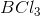II.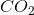III.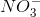IV.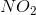IV only

I and II

I, II, and IV

II and III

I and IV

I and IV

Explanation:

BCl3 only has six electrons around boron, while NO2 (with an odd number of electrons) would have only 7 electrons around the central nitrogen.

### Example Question #50 : Compounds And Molecules

Which of the following elements will have two unpaired valence electrons?

Fluorine

Carbon

Beryllium

Neon

Nitrogen

Carbon

Explanation:

Lewis dot diagrams can help us keep track on how the valence electrons will disperse themselves among orbitals in the atom. When drawing these diagrams, it is important to keep two things in mind.

1. The s orbital will be filled before the p orbital begins to be filled.

2. Electrons will never pair in the same orbital if an orbital of equal energy is unoccupied.

Knowing these two facts, we can predict which of the following elements will have two unpaired electrons.

Fluorine will have seven valence electrons, meaning that only one orbital will not be completely filled.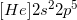Beryllium only has two valence electrons, but they will both be found in the 2s orbital, because it must be filled before the p orbitals can receive electrons.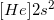Nitrogen will have five valence electrons, so two will be found in the 2s orbital. The other three will be present in their own p orbitals, meaning nitrogen has three unpaired electrons.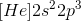Neon will have eight valence electrons, fully filling the 2s and 2p orbitals. It will have no unpaires electrons.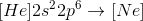Carbon has one less valence electron than nitrogen, meaning that it will have two unpaired electrons in the p orbitals.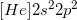### Example Question #45 : Compounds And Bonding

Which of the following is isoelectric with the cation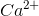?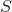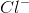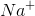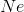Explanation:

Isoelectronic means having the same electron configuration (i.e. the same number of electrons).andboth have the same number of electrons (18), while sulfur has 16, phosphorous has 15, neon has 10, and the sodium cation has 10.

### Example Question #1 : Diagrams And Geometry

Which answer option correctly depicts the Lewis dot structure of sodium chloride?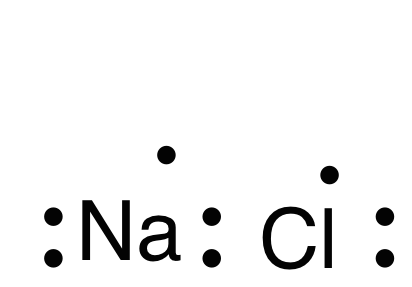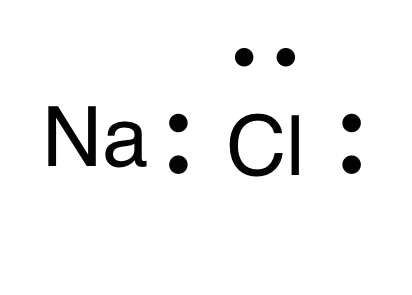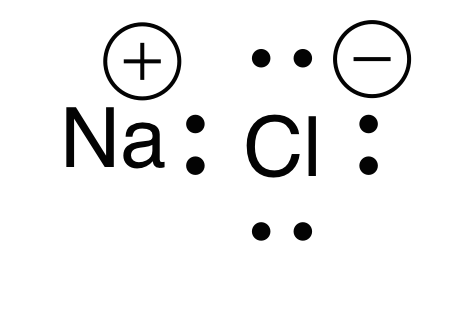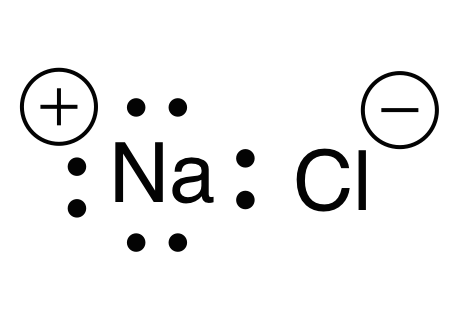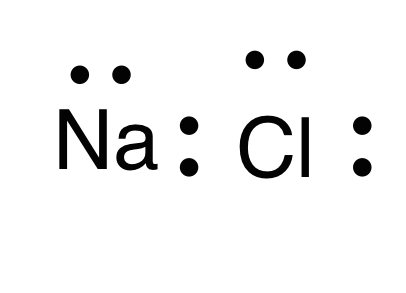Explanation:

When drawing a Lewis dot structure, we are always trying to reach an electron count where all atoms involved are stable and (usually) have full octets. We are also trying to estabilsh a structure in which we have the smallest formal charge possible. The general rule is first to draw out all of the elements involved and their valence electrons, then start piecing them together trying to reduce the formal charge and get all elements involved to an octet. There are a couple exceptions to the octet rule.

Sodium and chlorine form an ionic bond, meaning that one atom will donate an electron and the other will receive it. This gives each atom a charge. Chlorine has seven valence electrons, while sodium has one valence electron. For each atom to arrive at an octet, sodium will need to lose one electron and chlorine will need to gain one electron. This would give chlorine a negative charge, and sodium a positive charge.

Thus, the answer is a sodium with a positive charge (due to one lost electron) and a chlorine with eight electrons and a negative charge (due to one electron gained).

### Example Question #47 : Compounds And Bonding

What is the Lewis dot structure for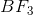?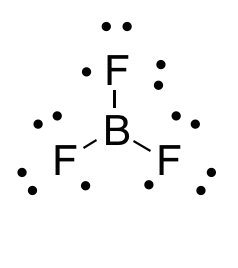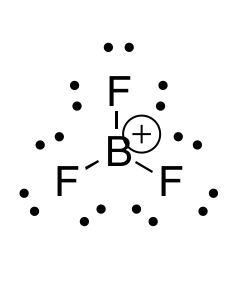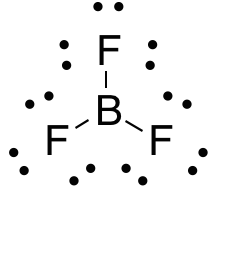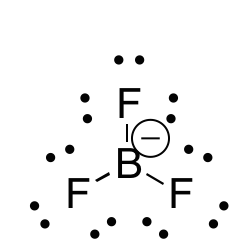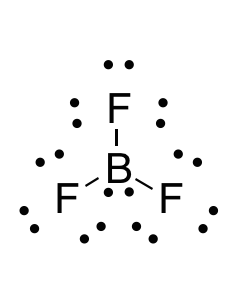Explanation:

When drawing a Lewis dot structure, we are always trying to reach an electron count where all atoms involved are stable and (usually) have full octets. We are also trying to estabilsh a structure in which we have the smallest formal charge possible. The general rule is first to draw out all of the elements involved and their valence electrons, then start piecing them together trying to reduce the formal charge and get all elements involved to an octet. There are a couple exceptions to the octet rule.

In this case, boron actually has an incomplete octet. Though there are resonance forms in which boron has a full octet, when you calculate the formal charge of these configurations it will not be zero.

### Example Question #1 : Other Molecular Concepts

Which of the following is an intrinsic physical property of a chemical substance?

Viscosity

Enthalpy

Mass

Heat

Viscosity

Explanation:

Intrinsic properties do not depend on the amount of a substance present. Mass, heat, and enthalpy are all dependent on the amount of substance.

### Example Question #2 : Other Molecular Concepts

Which of the following is NOT true of the law of constant composition?

It is what ensures that every sample of H2O will have 2 hydrogen atoms for every 1 oxygen atom.

It states that a given compound will contain the same elements in an identical mass ratio.

It can be applied to solutions.

It can be applied to pure samples.

It can be applied to solutions.

Explanation:

The law of constant composition can't be applied to solutions because it only applies to pure samples. Solutions are not pure samples, since they contain more than just 1 compound.

### Example Question #3 : Other Molecular Concepts

A compound that is formed in the mechanism of a reaction but does not appear in the overall reaction is referred to as a(n) __________.

product

catalyst

reactant

intermediate

intermediate

Explanation:

This is the very definition of an intermediate. It is formed in one step within the mechanism of the reaction, but then something else happens to it as the reaction continues to completion; thus, it does not show up in the overall reaction.

### Example Question #4 : Other Molecular Concepts

A compound is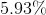hydrogen and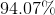oxygen by mass. What is the name and molecular formula of this compound?

Alcohol,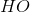Alcohol,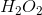Peroxide,Peroxide,None of these are correct

Peroxide,Explanation:

Knowing there is at least one hydrogen atom and one oxygen atom in this compound means the total molar mass must be at least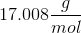. If we first assumeis equivalent to only 1 mole of hydrogen, we get a total molar mass of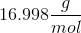. This number is too low to make logical sense.

Therefore, we must next assume there are 2 moles of hydrogen. Using this assumption, we can find the hypothetical molecular mass.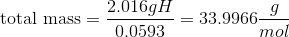Use this molecular mass to calculate the mass of oxygen.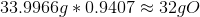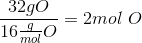Therefore, there must be 2 moles of hydrogen and 2 moles of oxygen, making the molecular formula.

This compound is called peroxide.

← Previous 1